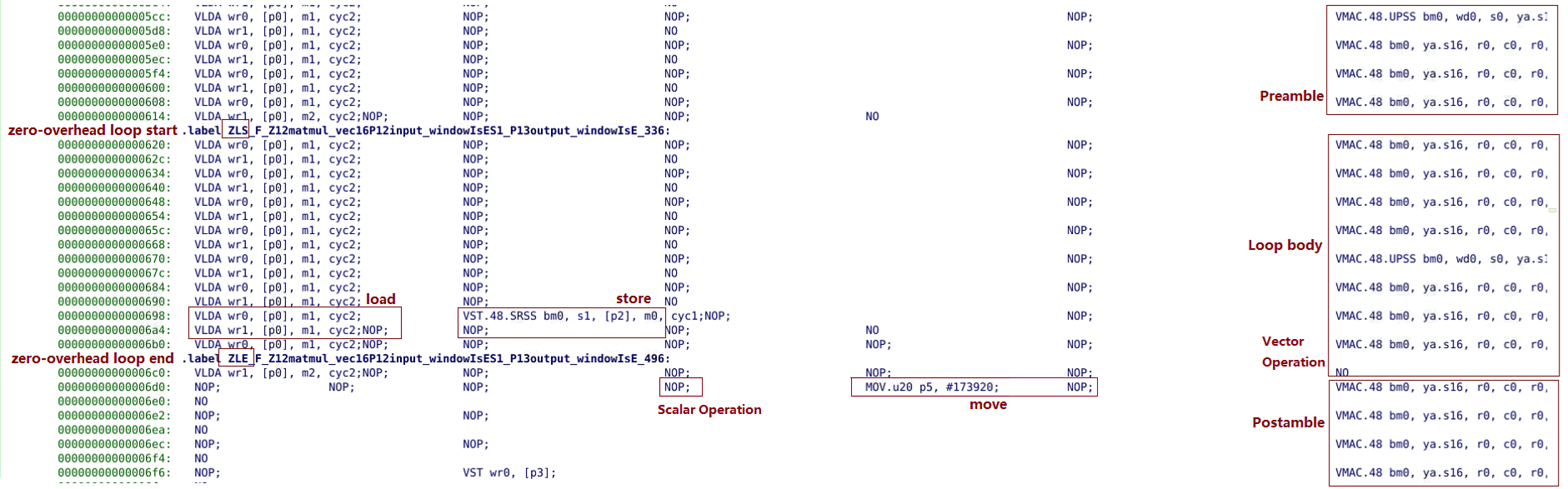# Loops - 2022.1 English

## AI Engine Kernel Coding Best Practices Guide (UG1079)

Document ID
UG1079
Release Date
2022-05-25
Version
2022.1 English

The AI Engine has a zero-overhead loop structure that does not incur any branch control overhead for comparison and branching thus reducing the inner loop cycle count. Pipelining allows the compiler to add pre-amble and post-amble so that the instruction pipeline is always full during loop execution. With a pipelined loop, a new iteration can be started before the previous one ends to achieve higher instruction level parallelism.

The following figure shows the assembly code of a zero-overhead loop. Note that two vector loads, one vector store, one scalar instruction, two data moves, and one vector instruction are shown in order in different slots.

Figure 1. Assembly Code of Zero-Overhead LoopThe following pragmas work together to direct the compiler to pipeline the loop and let it know that the loop will always be executed at least three times.

``````for (int i=0; i<N; i+=2)
chess_prepare_for_pipelining
chess_loop_range(3,)
``````

The ```chess_loop_range(<minimum>, <maximum>)``` tells the compiler that the corresponding loop is executed at least `<minimum>` times, and at most `<maximum>` times, where `<minimum>` and `<maximum>` are non-negative constant expressions, or can be omitted. When omitted, `<minimum>` defaults to 0, and `<maximum>` defaults to the maximum preset in the compiler. While `<maximum>` is not relevant for the pipeline implementation, `<minimum>` guides the pipeline implementation.

The `<minimum>` number defines how many loop iterations are executed at a minimum each time the loop is executed. The software pipeline is then tuned to allow at least that many iterations to execute in parallel if possible. It also determines that checking the boundaries for the loop is not necessary before the `<minimum>` number of iterations are executed.

The loop range pragma is not needed if the loop range is a compile time constant. In general, the AI Engine compiler reports the theoretical number best suited for optimum pipelining of an algorithm. If the range specification is not optimal, the compiler would issue a warning and suggest the optimal range. Towards that end, it is okay to initially set the `<minimum>` to one `[chess_loop_range(1,)]` and observe the theoretical best suited `<minimum>` being reported by the compiler.

``````Warning in "matmul_vec16.cc", line 10: (loop #39)
further loop software pipelining (to 4 cycles) is feasible with `chess_prepare_for_pipelining'
but requires a minimum loop count of 3
... consider annotating the loop with `chess_loop_range(3,)' if applicable,
... or remove the current `chess_loop_range(1,)` pragma``````

At this point, you can choose to update the `<minimum>` number to the reported optimum.

This second part of the pipeline implementation can be a reason for potential deadlocks in the AI Engine kernels if the actual `<minimum>` number of iterations is not reached. For this reason, you must ensure that the number of iterations is always at least the number specified in the `chess_loop_range` directive.

Loop carried dependencies impact the vectorization of code. If an inner loop dependency cannot be removed, a strategy to step out a level and manually unroll where there are (effectively) multiple copies of the inner loop running in parallel.

When looping though data, to increment or decrement by a specific offset, use the `cyclic_add` intrinsic function for circular buffers. The `fft_data_incr` intrinsic function enables the iteration of the pointer that is the current target of the butterfly operation. Using these functions can save multiple clock cycles over coding the equivalent functionality in standard C. Depending on the data types, you might need to cast parameters and return types.

The following example uses `fft_data_incr` intrinsic when operating on a matrix of real numbers.

``pC = (v8float*)fft_data_incr( (v4cfloat*)pC, colB_tiled, pTarget);``

Try to avoid sequential load operations to fill a vector register completely before use. It is best to interleave loads with MAC intrinsic functions, where the current MAC and next load can be done in the same cycle.

``````acc = mul4_sym(lbuff, 4, 0x3210, 1, rbuff, 11, coeff, 0, 0x0000, 1);
lbuff = upd_w(lbuff, 0, *left);
acc = mac4_sym(acc, lbuff, 8, 0x3210, 1, rbuff, 7, coeff, 4, 0x0000, 1);
``````

In certain use cases loop rotation, which rotates the instructions inside the loop, can be beneficial. Instead of loading data into a vector at the start of a loop, consider loading a block of data for the first iteration before the loop, and then for the next iteration near the end of the loop. This will add additional instructions but shorten the dependency length of the loop which helps to achieve an ideal loop with a potentially lower loop range.

``````// Load starting data for first iteration
sbuff = upd_w(sbuff, 0, window_readincr_v8(cb_input)); // 0..7

for ( int l=0; l<LSIZE; ++l )
chess_loop_range(5,)
chess_prepare_for_pipelining
{
sbuff = upd_w(sbuff, 1, window_readincr_v8(cb_input)); // 8..15
acc0 = mul4_sym(     sbuff,5 ,0x3210,1 ,12 ,coe,4,0x0000,1);

sbuff = upd_w(sbuff, 2, window_readdecr_v8(cb_input)); // 16..23
acc0 = mac4_sym(acc0,sbuff,1 ,0x3210,1 ,16,coe,0,0x0000,1);
acc1 = mul4_sym(     sbuff,5 ,0x3210,1 ,20,coe,0,0x0000,1);
window_writeincr(cb_output, srs(acc0, shift));
// Load data for next iteration
sbuff = upd_w(sbuff, 0, window_readincr_v8(cb_input)); // 0..7
acc1 = mac4_sym(acc1,sbuff,9,0x3210,1,16,coe,4,0x0000,1);
window_writeincr(cb_output, srs(acc1, shift));
}``````